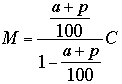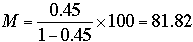SEARCH HOMEMath Central Quandaries & QueriesQuestion from Tonya: I'm trying to figure out the formula for the following, I have a product that I want to receive a certain profit, but with this product I also have to pay a percentage of commission to someone. I need to know how to calculate my new selling price taking into account the markup percentage, but I don't want my cut to be lowered. I have calculated the markup percentage to get my new selling price with the commission, but it lowers my cut once I pay the commission and I don't want it to do that. ThanksHi Tonya,

I'll show you want I think you want but let me know if I have misinterpreted your question.

You have a product you want to sell, your cost is C and you want to determine the markup M, both in dollars. You have to take into account a commission and your profit, both of which are percentages of what you charge your customer, (C + M) dollars. Suppose that the commission is a% of (C + M) and your profit is p% of (C + M). Hence the commission will be

(C + M) × a/100 dollars

(C + M) × p/100 dollars.

If you subtract the commission and your profit from what you charge your customer you should obtain your cost. That is

(C + M) - (C + M) × a/100 - (C + M) × p/100 = C

Solving this for M I getFor example if C = \$100, a = 15% and p = 30% thendollars.

PennyMath Central is supported by the University of Regina and The Pacific Institute for the Mathematical Sciences.